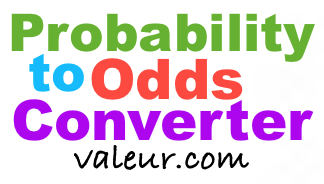Probability to Odds ConverterOur Probability to Odds Converter converts Percent Probability to Odds.

Below, please enter your Percent Probability that you want converted and press "To Odds".

This converter does more than just give you the answer! It will teach you too! When you enter a Percent Probability above to convert to Odds, we will show you step-by-step how we calculated the answer.

Odds To Probability Converter
Go here if you need to convert from Odds to Probability instead.

Here are some Probability to Odds conversions you may find interesting:

Convert 10 Percent Probability to Odds

Convert 25 Percent Probability to Odds

Convert 40 Percent Probability to Odds

Convert 60 Percent Probability to Odds